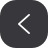# 关于哈密顿路是否存在的遍历算法

## 二、基本术语

：路是一条途径，途径中的各个顶点不同

：对于无向图，即为每个顶点上边的数目

## 三、问题及其衍生问题• 对于偶数阶的此类图，最外边一圈无论那个点缺失均存在哈密顿路
• 对于奇数阶的此类图，只有最外边一圈中缺失的点位于那条边的相对偶数位置不存在哈密顿路，奇数位置也是存在哈密顿路

## 四、算法思想

### 1.定理证明刨析

**设图G的邻接矩阵为 A A A n A^n 的元素 A n [ i ] [ j ] A^n[i][j] 等于由顶点 i i 和顶点 j j 的长度为n的路径的数目。**这个定理的证明理解是算法能够成功实现的关键，定理的本质上就是模拟寻路的过程，比如 A [ i ] [ j ] A[i][j] 代表第 i i 个点到第 j j 点是否相连，将 A ∗ A A*A 的元素展开，例如 A 2 [ 1 ] [ 1 ] = a 11 ∗ a 11 + a 12 ∗ a 21 . . . A^2 = a_{11}*a_{11} + a_{12}*a_{21}... 后面就不再展开了，每一个式子本质上是一条路径， a 11 ∗ a 11 a_{11}*a_{11} 代表迹:1 1 1, a 12 ∗ a 21 a_{12}*a_{21} 代表迹:1 2 1,以此类推，由于矩阵的特性，所以每次成一个矩阵代表走一步的意思，如果这些式子不进行合并的话，那么下一次 A 3 [ 1 ] [ 1 ] = a 11 ∗ a 11 ∗ a 11 + a 12 ∗ a 21 ∗ a 11 . . . A^3 = a_{11}*a_{11}*a_{11} + a_{12}*a_{21}*a_{11}... ，如果你继续写下去就会发现这个本质上就是一个探索全部路线的一个过程，这个定理的巧妙之处就是它没有记录之前路径的信息，所以它的运算非常高效，它仍然可以作为此题排除点的一个好办法，这个运算无论是时间还是空间复杂度，如果不加干预将达到可怕的 o ( n n ) o(n^n) ,这不是我们想看到的，定理对这种情况进行整合，使得每次进行矩阵运算时的时间和空间复杂度都是一样的，但它抛掉了所有的信息即路径的信息，所以基于以上定理，稍微做了一点点改进，保留路径信息同时尽量使得算法高效。

### 2.改进后的算法

• 保留矩阵的运算过程，尽量少做修改，保持理论上的可行性
• 最后的运算结果包含路径信息

#### 2.1 重定义类型

supperInt：这里重新定义了一种类型，包含一个int类型的数值，用于路径数目的计数，一个线性表，存放当前位置路径的集合，这里的类型相当于矩阵中的一个int型的元素，只是这里携带了路径信息。

commonInt：这是对于矩阵计算中需要下一个元素的当前下标设计的，这个类型用于辅助正常矩阵中元素的计算，包括一个int类型的数值和一个下标（也是int类型的）

#### 2.2 重载运算符

• 乘法运算符的重载

左操作数：supperInt

右操作数：commonInt

这里出现的场景为矩阵运算里面具体元素的相乘，不过你可能会有疑问，两个操作数类型为啥不同，这个稍后解释

• 加法重载运算符

左操作数：supperInt

右操作数：supperInt

这个也是矩阵运算过程中出现的加法，然后进行重载

• 矩阵乘法的重载

左操作数：vector

右操作数：int n [ d i m e ] [ d i m e ] n[dime][dime]

一个是列向量一个是矩阵

### 3.具体运算流程### 4.关键算法

• supperInt有一个value值，负责存储路径数目
• supperInt中的一个线性表负责存储路径
• commonInt中的value负责存储值，这个值即原始邻接矩阵中的值
• commonInt中的index代表下一步的去向，即到那个点，value为0代表到不了，反之代表可以到

## 五、算法实现步骤

#pragma once
#include<vector>
#include<iostream>
#include <algorithm>
#define dime 24
using namespace std;


### 1.类型定义

typedef struct commonInt {
//具体值
//这里取值只有0和1
int value = 0;

//下标
int index = 0;
}commonInt;

class supperInt {
public:
//value为路径数目
int value;

//具体路径
vector<vector<int>> path;
supperInt() {
this->value = 0;
}

//重载的两个友元函数
friend supperInt operator + (supperInt s1, supperInt s2);
friend supperInt operator * (supperInt s, commonInt c);
};


### 2.加法重载

//加法重载，本质上是线性表合并
supperInt operator+(supperInt s1, supperInt s2)
{
supperInt s3;
s3.path.insert(s3.path.end(), s1.path.begin(), s1.path.end());
s3.path.insert(s3.path.end(), s2.path.begin(), s2.path.end());
s3.value = s1.value + s2.value;
return s3;
}


### 3.乘法重载

//乘法重载，主要是排除不符合的路径
supperInt operator*(supperInt s, commonInt c)
{
//对于值为0的快速排除，提高运算速度
if ((c.value == 0) || (s.value == 0)) {

//释放内存操作
s.path.clear();
s.path.swap(s.path);
s.value = 0;
}
else {
//一个个遍历检查，遇到重复的直接排除掉，并销毁内存
for (vector<vector<int>>::iterator it = s.path.begin(); it != s.path.end();) {

//find函数是库<algorithm>中的一个外部函数，用于查找值
vector<int>::iterator result = find((*it).begin(), (*it).end(), c.index);
if(result == (*it).end()){
(*it).push_back(c.index);

//循环内部++避免野指针
it++;
}
else {

//不符合的移除
//然后逻辑上的value值--
it = s.path.erase(it);
s.value--;
}
}
}
return s;
}


### 4.矩阵乘法重载

//矩阵乘法重载，这里需要注意的就是需要重新创建一个vector防止原数组丢失
//局限性就是n数组必须指定大小
vector<supperInt> operator *(vector<supperInt>& s1, int n[dime][dime]) {

//复制一个s2保持运算过程中数组的不变性
//这里复制采用copy性能略优于直接复制
vector<supperInt> s2;
s2.resize(s1.size());
copy(s1.begin(), s1.end(), s2.begin());

for (int i = 0; i < dime; i++) {
supperInt sum;
for (int j = 0; j < dime; j++) {

//对于矩阵中的元素包装成commonInt类型
//利用天然的数组下标节省内存
commonInt t;
t.index = i;
t.value = n[j][i];

//利用上面的运算符重载进行计算
sum = sum + s2[j] * t;
}
//第i个矩阵运算的结果
//这里会发生改变，故上述使用了一个临时数组
s1[i] = sum;
}
return s1;
}


### 5.主函数文件#### 5.1 初始化

//初始化一维数组
#define dim 24
#define step 23

vector<supperInt> target(dim);

//压入第一个初始路径
vector<int> ss;
ss.push_back(0);
ss.push_back(1);
target.path.push_back(ss);
target.value = 1;

//初始化矩阵
int A = { 0 };
A[ 1] = 1;
A[ 2] = 1; A[ 5] = 1;
A[ 3] = 1; A[ 6] = 1;
A[ 4] = 1; A[ 7] = 1;
A[ 8] = 1;
A[ 6] = 1; A[ 10] = 1;
A[ 7] = 1; A[ 11] = 1;
A[ 8] = 1; A[ 12] = 1;
A[ 13] = 1;
A[ 10] = 1; A[ 14] = 1;
A[ 11] = 1; A[ 15] = 1;
A[ 12] = 1; A[ 16] = 1;
A[ 13] = 1; A[ 17] = 1;
A[ 18] = 1;
A[ 15] = 1; A[ 19] = 1;
A[ 16] = 1; A[ 20] = 1;
A[ 17] = 1; A[ 21] = 1;
A[ 18] = 1; A[ 22] = 1;
A[ 23] = 1;
A[ 20] = 1;
A[ 21] = 1;
A[ 22] = 1;
A[ 23] = 1;

//下三角的赋值
for (int i = 0;i < dim;i++) {
for (int j = 0; j < i; j++) {
A[i][j] = A[j][i];
}
}


#### 5.2 开始计算

//开始逻辑计算
//这里的步数可以调整，正常时步数-1次循环，判断是否有哈密顿路，第一步在初始化中已经完成了
for (int i = 0; i < step - 1; i++) {
target = target * A;
}


#### 5.3 展示结果

//计算解决方案数
int sum = 0;
for (int i = 0; i < dim; i++) {
sum = sum + target[i].value;
}

cout << "总的解决方案数:" << sum << endl;
cout << "每个解决方案所用的步数:" << step << "步" << endl;
for(int i= 0;i < dim;i++){

cout << "从1结点到达" << (i + 1) << "结点的解决方案数目：" << target[i].value << "\n";

for (int j = 0; j < target[i].path.size(); j++) {
for (auto it = target[i].path[j].cbegin(); it != target[i].path[j].cend(); it++)
{
cout << (*it + 1) << " ";
}
cout << "\n";
}
cout << "\n";
}## 八、总结

“种一棵树的最好时间是十年前，其次是现在。”，确实十年过去了，心中的萌芽已然成为参天大树，虽未能真正完成问题的解决，但最初的问题已经解决，这样就足够了，希望大家也能够有那么一件一生值得做的事情，做好，这样就足够了。

07-081187
09-28411
06-21690
07-035133
05-111461
08-301234
07-313067
07-304万+
01-101万+
04-16496
11-222万+
04-192808
11-062万+
07-011万+
12-242万+
07-08643
04-122828
07-303365

### “相关推荐”对你有帮助么？

•非常没帮助
•没帮助
•一般
•有帮助
•非常有帮助被折叠的  条评论 为什么被折叠?到【灌水乐园】发言wresource

¥2 ¥4 ¥6 ¥10 ¥20余额支付 (余额：-- )扫码支付获取中扫码支付点击重新获取扫码支付1.余额是钱包充值的虚拟货币，按照1:1的比例进行支付金额的抵扣。
2.余额无法直接购买下载，可以购买VIP、C币套餐、付费专栏及课程。余额充值